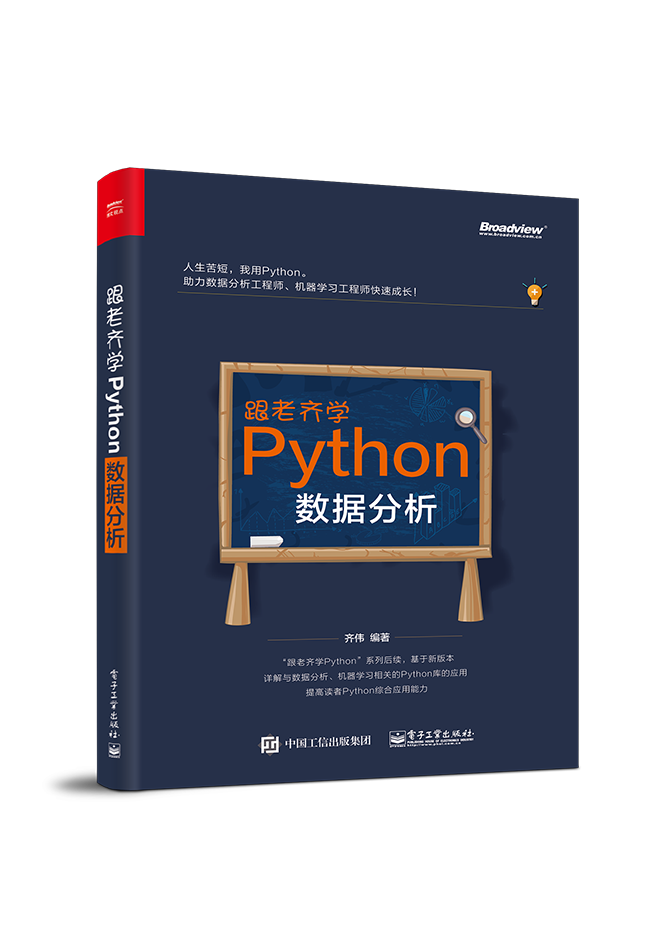## 用Python实现各类数学符号运算$$\frac{1}{N}\sum_{i=1}^N(y_i-\hat{y_i})^2$$

### 索引

$$x_i$$

$$X_{ij}$$

### 求和

$$\sum_{i=1}^Nx_i$$

### PI

$$\prod_{i=1}^Nx_i$$

### 管道符

#### 绝对值

$$|X|$$

#### 范数

$$||X||$$

### Epsilon

$$3\in X$$

### 函数

$$f:X \to Y$$

$$f:R \to R$$

R意味着输入输出都是实数，比如整数、浮点数、无理数、有理数等。Python中当然可以表示：

$$f:R^d \to R$$

$R^d$意味着实数组成的d维度向量（译者注：实数域的d维子空间）。

### 张量

#### 转置

$$X^T$$

#### 乘法

$$X \bullet Y$$

#### 点积

$$XY$$
$$X.Y$$

#### 有帽子的符号

$$\hat{x}$$

### 感叹号

$$x!$$